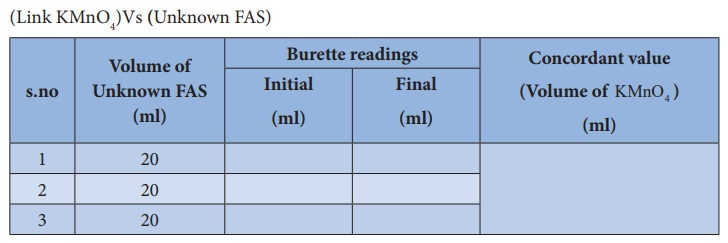Home | | Chemistry 12th Std | Estimation of Ferrous Ammonium Sulphate (FAS)

# Estimation of Ferrous Ammonium Sulphate (FAS)

To estimate the amount of ferrous ammonium sulphate (FAS) dissolved in 1500 ml of the given unknown solution volumetrically.

Estimation of Ferrous Ammonium Sulphate (FAS)

## Aim :

To estimate the amount of ferrous ammonium sulphate (FAS) dissolved in 1500 ml of the given unknown solution volumetrically. For this you are given with a standard solution of ferrous sulphate ( FeSO4 )of normality 0.1024 N and potassium permanganate solution as link solution.

## Principle:

Oxidation : 5 Fe2+   5 Fe3+ + 5e

Reduction: 5Fe2+ + MnO4 + 8H+  → 5Fe3+ + Mn2+ + 4H2O

Overall reaction: 5Fe2+ + MnO4 + 8H+     → 5Fe3+ + Mn2+ + 4H2O

Short procedure:## Procedure :

### Titration–I

Burette is washed with water, rinsed with KMnO4 solution and filled with same solution up to the zero mark. Exactly 20 ml of standard FeSO4 solution is pipetted out into the clean, washed conical flask. To this solution, approximately 20ml of 2N sulphuric acid is added. This mixture is titrated against KMnO4 Link solution from the burette. KMnO4 is added drop wise till the appearance of permanent pale pink colour. Burette reading are noted, the same procedure is repeated to get concordant values.

### Titration –I## Calculation :

Volume of KMnO4 (link) solution  V1 =     ml

Normality KMnO4 (link) solution     N1      =       ?N

Volume of standard FeSO4 solution V2      =       20 ml

Normality of standard FeSO4 solution       N2      =       0.1024 N

## According to normality equation:

According to normality equation: V1× N1 = V2 × N2

N1 = V2 × N2 / V1Normality of KMnO4 (link) solution (N1) = ----------X--------- N

### Titration–II

(Unknown FAS) Vs (Link KMnO4 )

Burette is washed with water, rinsed with KMnO4 solution and filled with same KMnO4 solution up to the zero mark. Exactly 20 ml of unknown FAS solution is pipetted out into the clean, washed conical flask. To this FAS solution approximately 20ml of 2N sulphuric acid is added. This mixture is titrated against KMnO4 Link solution from the burette. KMnO4 is added drop wise till the appearance of permanent pale pink colour. Burette reading is noted and the same procedure is repeated to get concordant values.

### Titration –II## Calculation :

Volume of Unknown FAS solution V1  = 20ml

Normality of Unknown FAS solution N1 = N

Volume of KMnO4 (link) solution V2 = ml

According to normality equation: V1× N1 = V2 ×

N2 N1 = V2  × N2 / V1N1= ------------------------ N

The normality of unknown FAS solution   = --------Y---N

## Weight calculation:

The amount of FAS dissolved in 1 lit of the solution = (Normality) x (equivalent weight)

The amount of FAS dissolved in 1500 ml of the solution = Normality × equivalentweight × 1500 / 1000= Y × 392  × 1500 / 1000

= g

## Report :

The amount of FAS dissolved in 1500 ml of the solution = g

Tags : Volumetric Analysis | Chemistry Practical Laboratory Experiment , 12th Chemistry : Practicals
Study Material, Lecturing Notes, Assignment, Reference, Wiki description explanation, brief detail
12th Chemistry : Practicals : Estimation of Ferrous Ammonium Sulphate (FAS) | Volumetric Analysis | Chemistry Practical Laboratory Experiment